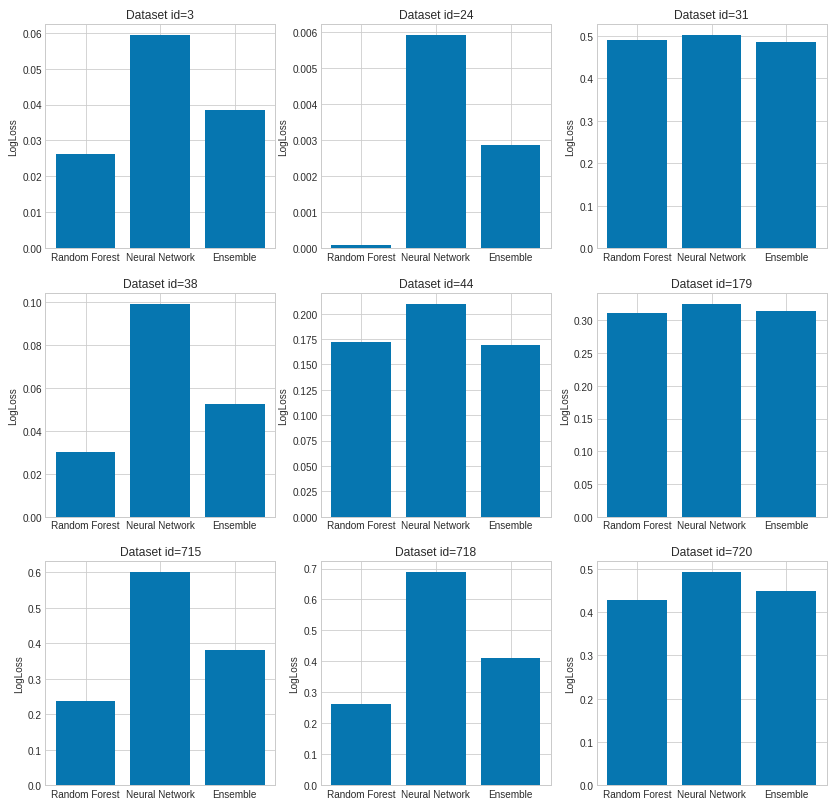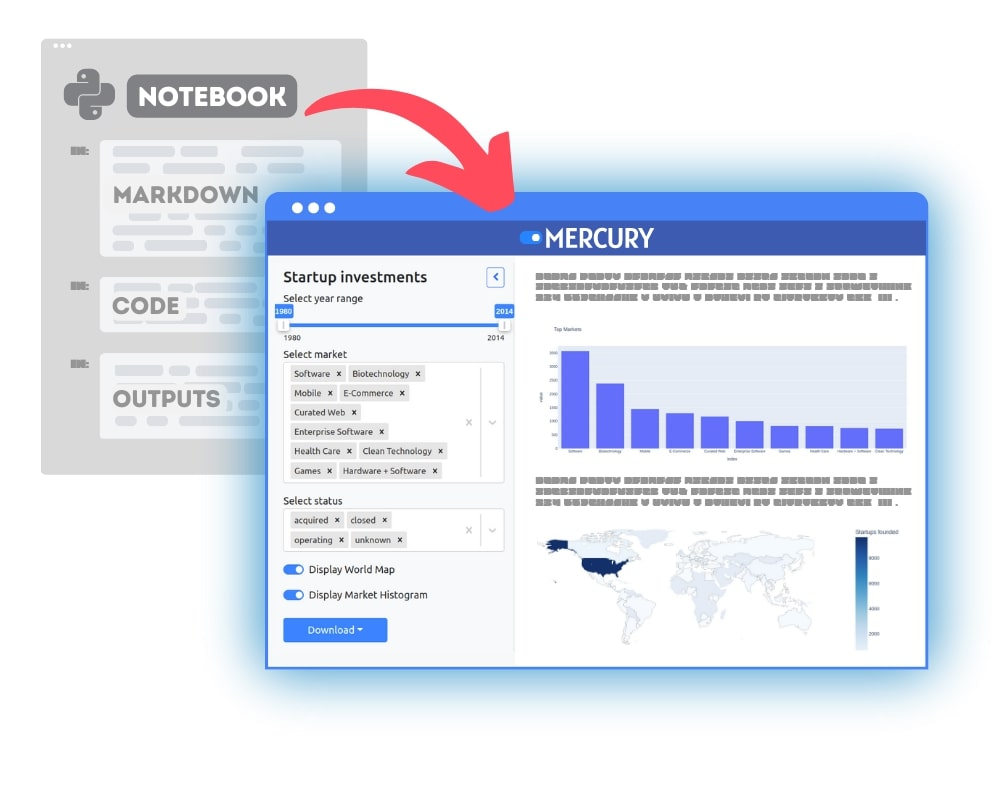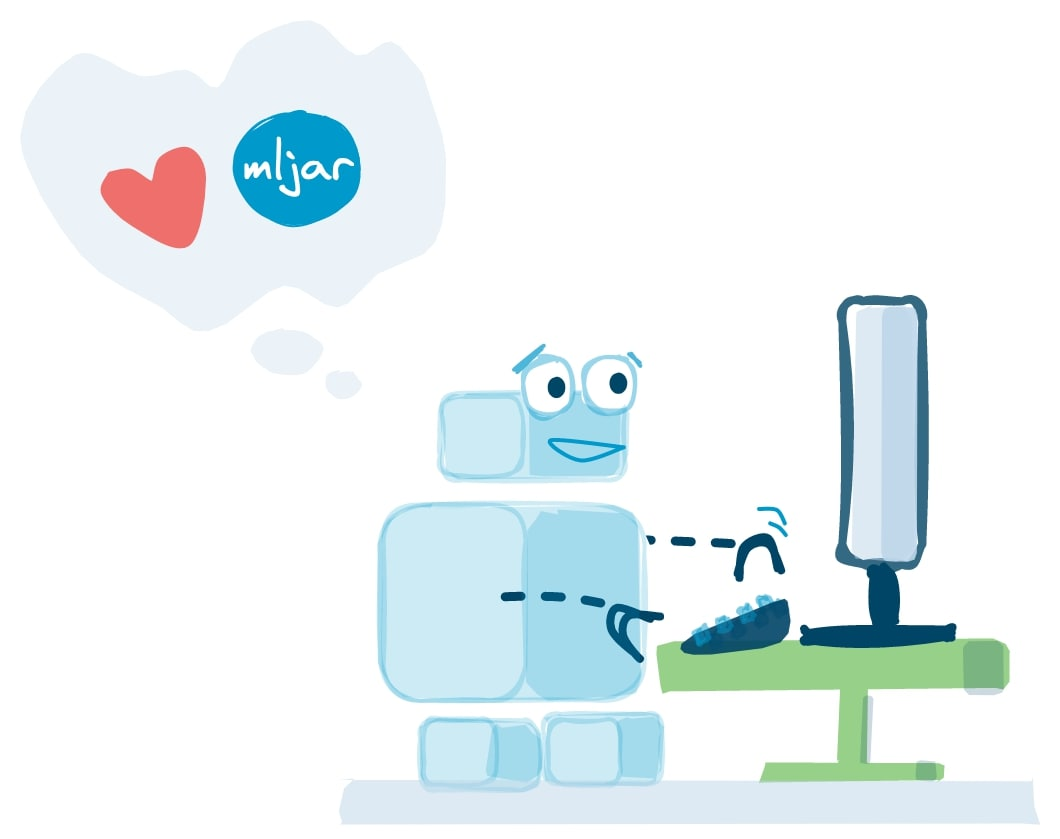Which is better: Random Forest or Neural Network? This is a common question, with a very easy answer: it depends :) I will try to show you when it is good to use Random Forest and when to use Neural Network.

First of all, Random Forest (RF) and Neural Network (NN) are different types of algorithms. The RF is the ensemble of decision trees. Each decision tree in the ensemble process the sample and predicts the output label (in case of classification). Decision trees in the ensemble are independent. Each can predict the final response. The Neural Network is a network of connected neurons. The neurons cannot operate without other neurons - they are connected. Usually, they are grouped in layers and process data in each layer and pass forward to the next layers. The last layer of neurons is making decisions.

The Random Forest can only work with tabular data. (What is tabular data? It is data in a table format). On the other hand, Neural Network can work with many different data types:

• tabular data
• images (the NN become very popular after beating image classification benchmarks, for more details please read more about Convolutional Neural Networks (CNN))
• audio data (also handled with CNN)
• text data - can be handled by NN after preprocessing, for example with bag-of-words. In theory, RF can work with such data as well, but in real-life applications, after such preprocessing, data will become sparse and RF will be stuck.

OK, so now you have some intuition, that when you deal with images, audio or text data, you should select NN.

In the case of tabular data, you should check both algorithms and select the better one. Simple. However, I would prefer the Random Forest over Neural Network, because there are easier to use. I’ll show you why.

### Random Forest vs Neural Network - data preprocessing

In theory, the Random Forest should work with missing and categorical data. However, the sklearn implementation doesn’t handle this (link1, link2). To prepare data for Random Forest (in python and sklearn package) you need to make sure that:

• there are no missing values in your data
• convert categoricals into numbers

Data preprocessing for Neural Networks requires filling missing values and converting categoricals into numbers. What is more, there is a need for feature scaling. In the case of different ranges of features, there will be problems with model training. If you don’t scale features into the same ranges then features with larger values will be treated as more important in the training, which is not desired. What is more, the gradients values can explode and the neurons can saturate which will make impossible to train NN. To conclude, for Neural Network training, you need to do the following preprocessing:

• fill missing values
• convert categoricals into numericals
• scale features into the same (or at least similar) range

Keep in mind, that all preprocessing that is used for preparing training data should be used in production. For NN you have more steps for preprocessing so more steps to implement in the production system as well!

## Random Forest vs Neural Network - model training

Data is ready, we can train models.

For Random Forest, you set the number of trees in the ensemble (which is quite easy because of the more trees in RF the better) and you can use default hyperparameters and it should work.

You need some magic skills to train Neural Network well :)

• you need to define the NN architecture. How many layers to use, usually 2 or 3 layers should be enough. How many neurons to use in each layer? What activation functions to use? What weights initialization to use?
• Architecture ready. Then you need to choose a training algorithm. You can start with simple Stochastic Gradient Descent, but there are many others (RMSprop, Adagrad, Adam, Adadelta … take a look at optimizers in Keras). Let’s go with ‘simple’ SGD: you need to set learning rate, momentum, decay. Not enough hyperparameters? You need to set a batch size as well (batch - how many samples to show for each weights update).

You know what is funny. That each of the NN hyperparameters mentioned above can be critical. For example, you set too large learning rate or not enough neurons in second hidden-layer and your NN training will be stuck in a local minimum. Uhhh …

## The empirical example

Stop talking, show me the results! OK, let’s train models.

I will train Random Forest and Neural Network on 9 datasets from OpenML.org data repository.

The dataset description:

id title rows cols
3 kr-vs-kp 3196 37
24 mushroom 8124 23
31 credit-g 1000 20
38 sick 3772 30
44 spambase 4601 58
715 fri_c3_1000_25 10000 25
718 fri_c4_1000_100 10000 100
720 abalone 4177 9

As you see, datasets I’m using are rather small, up to a few thousand rows and several columns. I want to have small data for quick example nonetheless they cover some range of possible use cases. You can access each dataset by id from openml.org. I will use 70% of the data for training and the rest for testing with a random split.

For Random Forest and Neural Network training, I have used my open source AutoML package mljar-supervised. I prefer to use AutoML approach here because it does data preprocessing for me and tune hyperparameters.

``````result = {}

for dataset_id in [3, 24, 31, 38, 44, 179, 715, 718, 720]:

result[dataset_id] = {"logloss":{"rf":[], "nn": [], "ensemble": []},
"f1":{"rf":[], "nn": [], "ensemble": []}}

x_cols = [c for c in df.columns if c != 'target']
X = df[x_cols]
y = df['target']
for repeat in range(5):
seed = 1707+repeat
X_train, X_test, y_train, y_test = \
sklearn.model_selection.train_test_split(X, y, test_size = 0.3, random_state=seed)

automl = AutoML(
total_time_limit=10*60, # 10 minutes
algorithms=["RF"],
train_ensemble=False,
verbose=False,
)
automl.fit(X_train, y_train)
y_predicted_rf = automl.predict(X_test)
result[dataset_id]["logloss"]["rf"] += [log_loss(y_test, y_predicted_rf['p_1'])]
result[dataset_id]["f1"]["rf"] += [f1_score(y_test, y_predicted_rf['label'])]

automl_nn = AutoML(
total_time_limit=10*60, # 10 minutes
algorithms=["NN"],
train_ensemble=False,
verbose=False,
)
automl_nn.fit(X_train, y_train)
y_predicted_nn = automl_nn.predict(X_test)
result[dataset_id]["logloss"]["nn"] += [log_loss(y_test, y_predicted_nn['p_1'])]
result[dataset_id]["f1"]["nn"] += [f1_score(y_test, y_predicted_nn['label'])]

y_predicted_ens = (y_predicted_rf + y_predicted_nn) / 2.0
result[dataset_id]["logloss"]["ensemble"] += [log_loss(y_test, y_predicted_ens['p_1'])]
``````

In the end, I am computing simple ensemble as the average of Random Forest and Neural Network predictions.

It is worth to mention about details of training NN with AutoML. Below are hyperparameters of NN that are selected in AutoML:

``````NeuralNetworkLearnerBinaryClassificationParams = {
"dense_layers": [1, 2, 3],
"dense_1_size": [4, 8, 16, 32, 64, 128],
"dense_2_size": [4, 8, 16, 32, 64],
"dense_3_size": [4, 8, 16, 32],
"dropout": [0, 0.25, 0.5, 0.75],
"learning_rate": [0.005, 0.01, 0.05, 0.1, 0.2],
"momentum": [0.85, 0.9, 0.95],
"decay": [0.0001, 0.001, 0.01],
}
``````

The AutoML is creating Neural Networks with 1, 2 or 3 layers and drawing the number of neurons. For training, the SGD is used with a batch size equal to 256.

The empirical results of comparison with 5-times repetition (the lower logloss the better):Are you surprised? Maybe it is possible the get better results by Neural Network but it will require a lot of manual tuning by the expert.

The next surprising thing is the results of simple ensemble average which slightly improves the final prediction only in 3 cases (datasets with id 31, 44, 179).

## Conclusions

When you have a dilemma which one to use, Random Forest or Neural Networks. You should decide based on the data type that you have. You should use the Neural Network for:

• images
• audio
• text

If you are going to work with tabular data, it is worth to check the Random Forest first because it is easier. The Random Forest requires less preprocessing and the training process is simpler. Therefore, it is simpler to use RF in the production system. If you are not satisfied with the model performance you should try to tune and train Neural Network. There are many hyperparameters which can be tuned in NN and if you have enough knowledge and experience you can obtain very good results with NN.

Good luck! :)### Convert Python Notebooks to Web Apps

We are working on open-source framework Mercury for converting Jupyter Notebooks to interactive Web Applications.### Articles you might find interesing